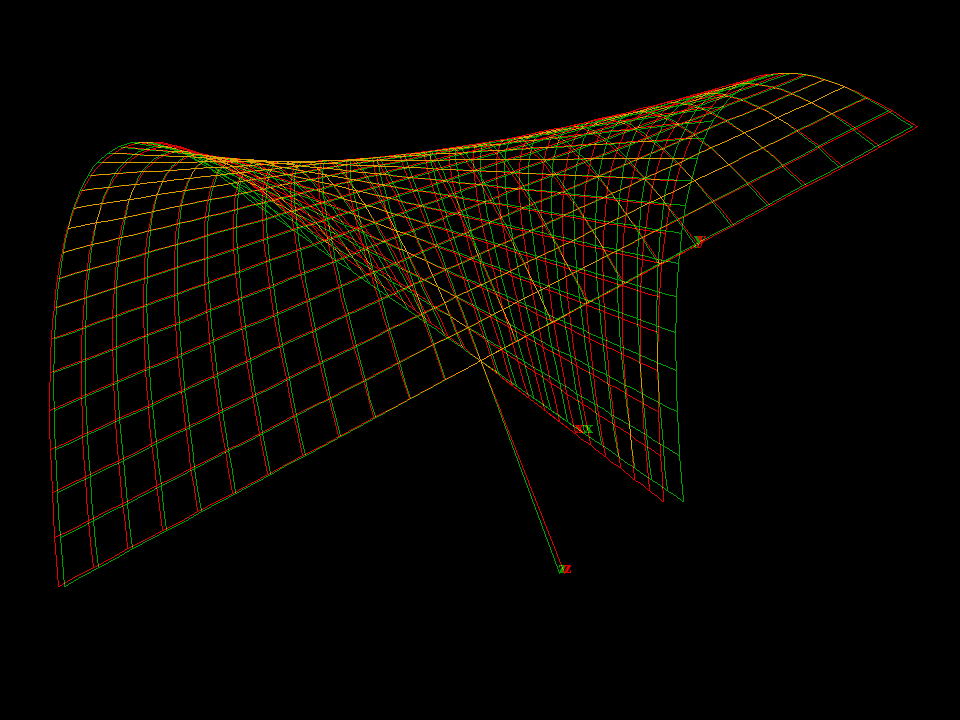# Whitney UmbrellaThe Whitney Umbrella is famous for its pinch-point singularity. In the animation a second such singularity is created.

The name umbrella comes from the fact that the implicit equation `x^2 - y^2 * z = 0` has the surface plus the z-axis (like a handle) as solution.Observe that the angle between the horizontal lines on the surface goes to zero as these lines approach the line with the singularity.

Strictly speaking, the Whitney umbrella is the algebraic surface defined by the implicit equation:

`x^2 - y^2*z == 0`

It has two algebraic components, a two-dimensional piece that is given parametrically by the equations:

```  x := u * v
y := u
z := v * v```

and a one-dimensional piece, namely the entire z-axis (the handle of the umbrella). The two-dimensional part is important in the theory of singularities. The point at the end of the line of self-intersections is a singularity of a type called a pinch point. This is a canonical representative of the only stable singularity for mappings from R^2 to R^3.

Get red/blue stereo glasses from amazon

RuledSurfaces.pdf